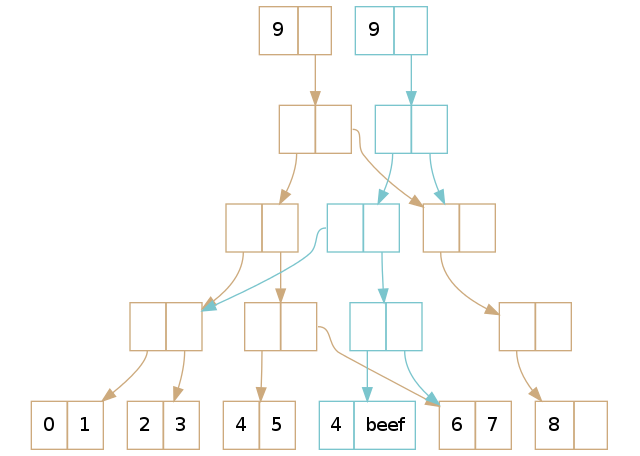# Immutability and Python

Introducing Pyrsistent

# Disclaimer

## What is immutability?

Something is immutable if it cannot be changed once created.

Lets keep it simple:

### Why immutability in programming?

• Cognitive offload for the developer
• Safe and simple invariance checking
• Safe and fast sharing, within and between threads
• Safe and fast reuse
• Hashable
• Runtime efficiency

### Why not?

• Runtime efficiency
• Habits
• Language support

## Immutability classes (WAT?)

• Immutable by convention
• Apparent immutability
• True immutability

## Base types

``````>>> x = 17
>>> y = 17
>>> x is y
True
>>> y = 1700
>>> x = 1700
>>> x is y
False``````

Numbers and strings are immutable

## Collections

tuple, frozenset and namedtuple, that's about it...

``````>>> tuple() is tuple()
True
>>> list() is list()
False``````

Gets messy and inefficient when you want to evolve the content

``````>>> t1 = (1, 2, 3, 4, 5)
>>> t2 = t1[:2] + tuple(17) + t1[3:]
# or
>>> l1 = list(t1)
>>> l1 = 17
>>> t2 = tuple(l1)``````

## Enter Pyrsistent

Immutable collections that are easy to evolve

a.k.a. persistent data structures

a.k.a. functional data structures

# Pyrsistent eco systema = apparent immutability

c = immutability by convention

# Examples

``````>>> students = freeze([{'name': 'Ann', 'grade': 4.1}, {'name': 'Bob', 'grade': 2.1}])
>>> students_v2 = students.set_in((1, 'grade'), 1.9)
>>> students
>>> students_v2
>>> thaw(students_v2)
``````
``````>>> Point = pclass('x, y', name='Point')
>>> p = Point(1, 2)
>>> p2 = p.set(x=3)
>>> p
Point(x=1, y=2)
>>> p2
Point(x=3, y=2)``````
``````>>> v1 = pvector([1, 2, 3, 4])
>>> v1 == v(1, 2, 3, 4)
True
>>> v1
2
>>> v1[1:3]
pvector([2, 3])
>>> v3 = v1.set(1, 5)
>>> v3
pvector([1, 5, 3, 4])
>>> v1
pvector([1, 2, 3, 4])
>>> pvector(x + 1 for x in v1)
pvector([2, 3, 4, 5])
``````
``````>>> m1 = pmap({'a':1, 'b':2})
>>> m1 == m(a=1, b=2)
True
>>> m1['a']
1
>>> m1.b
2
>>> m1.items()
[('a', 1), ('b', 2)]
>>> m1.set('a', 3)
pmap({'a': 3, 'b': 2})
>>> m1
pmap({'a': 1, 'b': 2})
``````

## Structural reuse``````>>> v1 = v(0, 1, 2, 3, 4, 5, 6, 7, 8)
>>> v1
pvector([0, 1, 2, 3, 4, 5, 6, 7, 8])
>>> v2 = v1.set(5, 'beef')
>>> v2
pvector([0, 1, 2, 3, 4, 'beef', 6, 7, 8])
>>> v1
pvector([0, 1, 2, 3, 4, 5, 6, 7, 8])
``````

Get it:

Contribute:

Docs:

`pip install pyrsistent`

## Thank you!

#### Immutability and Python

By Tobias Gustafsson

# Immutability and Python

A brief introduction to Pythons take on (or rather lack of) immutability followed by a compressed description of Pyrsistent. Pyrsistent is a library that tries to provide some of the pieces missing in the Python standard library for convinient work with immutable data structures.

• 7,053